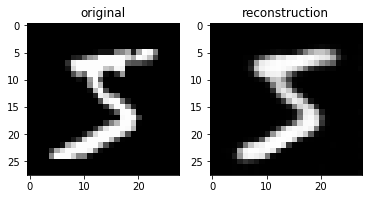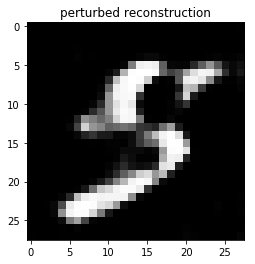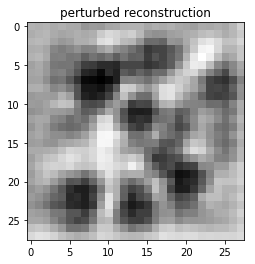# Generative Adversarial Networks¶

Thus far, we have discussed several generative models. A generative model learns the structure of a set of input data. In doing so, the model learns to generate new data that it has never seen before in the training data. The generative models we discussed were:

• an autoencoder
• an RNN used to generate text

A Generative Adversarial Network (GAN) is yet another example of a generative model. To motivate the GAN, let's first discuss the drawbacks of an autoencoder.

In :
%matplotlib inline

import matplotlib.pyplot as plt
import torch
import torch.nn as nn
import torch.nn.functional as F
import torch.optim as optim
import torch.utils.data
from torchvision import datasets, transforms


  0%|          | 0/9912422 [00:00<?, ?it/s]
Downloading http://yann.lecun.com/exdb/mnist/train-images-idx3-ubyte.gz to data/MNIST/raw/train-images-idx3-ubyte.gz

9920512it [00:01, 5125118.53it/s]

Extracting data/MNIST/raw/train-images-idx3-ubyte.gz

32768it [00:00, 444413.76it/s]
1%|          | 16384/1648877 [00:00<00:10, 151225.92it/s]
Downloading http://yann.lecun.com/exdb/mnist/train-labels-idx1-ubyte.gz to data/MNIST/raw/train-labels-idx1-ubyte.gz
Extracting data/MNIST/raw/train-labels-idx1-ubyte.gz

1654784it [00:00, 3035179.84it/s]
8192it [00:00, 182421.07it/s]

Extracting data/MNIST/raw/t10k-images-idx3-ubyte.gz
Extracting data/MNIST/raw/t10k-labels-idx1-ubyte.gz
Processing...
Done!


## Autoencoder Review¶

Here is the code that we wrote back in the autoencoder lecture. The autoencoder model consists of an encoder that maps images to a vector embedding, and a decoder that reconstructs images from an embedding.

In :
class Autoencoder(nn.Module):
def __init__(self):
super(Autoencoder, self).__init__()
self.encoder = nn.Sequential(
nn.Conv2d(1, 16, 3, stride=2, padding=1),
nn.ReLU(),
nn.Conv2d(16, 32, 3, stride=2, padding=1),
nn.ReLU(),
nn.Conv2d(32, 64, 7)
)
self.decoder = nn.Sequential(
nn.ConvTranspose2d(64, 32, 7),
nn.ReLU(),
nn.ReLU(),
nn.Sigmoid()
)

def forward(self, x):
x = self.encoder(x)
x = self.decoder(x)
return x


We trained an autoencoder model on the reconstruction loss: the difference in pixel intensities between a real image and its reconstruction. We won't run the entire training code today. Instead, we will load a model that was trained earlier.

In :
def train(model, num_epochs=5, batch_size=64, learning_rate=1e-3):
torch.manual_seed(42)
criterion = nn.MSELoss()
optimizer = torch.optim.Adam(model.parameters(), lr=learning_rate, weight_decay=1e-5)
outputs = []
for epoch in range(num_epochs):
for data in train_loader:
img, label = data
recon = model(img)
loss = criterion(recon, img)
loss.backward()
optimizer.step()

print('Epoch:{}, Loss:{:.4f}'.format(epoch+1, float(loss)))
outputs.append((epoch, img, recon),)
torch.save(model.state_dict(), "autoencoder%d.pt" % epoch)
return outputs

model = Autoencoder()
#outputs = train(model, num_epochs=5)

In :
# Choose a model to load -- after 2 epochs of training

Out:
IncompatibleKeys(missing_keys=[], unexpected_keys=[])

Let's take a look at one MNIST image from training, and its autoencoder reconstruction:

In :
original = mnist_data.unsqueeze(0)
emb = model.encoder(original)
recon_img = model.decoder(emb).detach().numpy()[0,0,:,:]

# plot the original image
plt.subplot(1,2,1)
plt.title("original")
plt.imshow(original, cmap='gray')

# plot the reconstructed
plt.subplot(1,2,2)
plt.title("reconstruction")
plt.imshow(recon_img, cmap='gray')

Out:
<matplotlib.image.AxesImage at 0x11b3b8ba8>The reconstruction is reasonable, but notice that the reconstruction is blurrier than the original image. If we perturb the embedding to generate a new image, we still should see this blurriness:

In :
# Run this a few times
x = emb + 10 * torch.randn(1, 64, 1, 1) # add a random perturbation

# reconstruct image and plot
img = model.decoder(x)[0,0,:,:]
img = img.detach().numpy()
plt.title("perturbed reconstruction")
plt.imshow(img, cmap='gray')

Out:
<matplotlib.image.AxesImage at 0x11f666550>In :
# Sidenote: Question from midterm
emb = torch.randn(1, 64, 1, 1) # random normal embedding
img = model.decoder(emb)[0,0,:,:]
img = img.detach().numpy()
plt.title("perturbed reconstruction")
plt.imshow(img, cmap='gray')
# End side note

Out:
<matplotlib.image.AxesImage at 0x11f79b828>The reason autoencoders tend to generate blurry images is because of the loss function that it uses. The use of MSELoss (mean square error loss) has an averaging effect. If the model learns that two possible values for a pixel is 0 and 1, then it will learn to predict a value of 0.5 for that pixel to minimize the mean square error. However, none of our training data might have a pixel intensity of 0.5 at that pixel! A human would easily tell the difference between a generated image and a real image.

But what would be a more appropriate loss function than the MSELoss? People have tried to come up with better loss functions, but it is difficult to construct a general enough loss function that is appropriate for all kinds of generation tasks. What we really want to do is learn a loss function!

The main idea is that generates images that fail to fool a human should also fail to fool a neural network trained to differentiate real vs fake images. We can use the prediction of this discriminator neural network to guide the training of our generator network.

## Generative Adversarial Network¶

A generative adversarial network (GAN) model consists of two models:

• A Generator network $G$ that takes in a latent embedding (usually random noise) and generates an image like those that exists in the training data
• A Discriminator network $D$ that tries to distinguish between real images from the training data, and fake images produced by the generator

In essense, we have two neural networks that are adversaries: the generator wants to fool the discriminator, and the discriminator wants to avoid being fooled. The setup is known as a min-max game.

Let's set up a simple generator and a discriminator to start:

In :
class Discriminator(nn.Module):
def __init__(self):
super().__init__()
self.model = nn.Sequential(
nn.Linear(28*28, 300),
nn.LeakyReLU(0.2, inplace=True),
nn.Linear(300, 100),
nn.LeakyReLU(0.2, inplace=True),
nn.Linear(100, 1)
)

def forward(self, x):
x = x.view(x.size(0), -1)
out = self.model(x)
return out.view(x.size(0))

class Generator(nn.Module):
def __init__(self):
super().__init__()
self.model = nn.Sequential(
nn.Linear(100, 300),
nn.LeakyReLU(0.2, inplace=True),
nn.Linear(300, 28*28),
nn.Sigmoid()
)

def forward(self, x):
out = self.model(x).view(x.size(0), 1, 28, 28)
return out


For now, both the Discriminator and Generator are fully-connected networks. One difference between these models and the previous models we've built is that we are using a nn.LeakyReLU activation.

Actually, you have seen leaky ReLU activations before, from the very beginning of the course, in assignment 1! Leaky ReLU is a variation of the ReLU activation that lets some information through, even when its input is less than 0. The layer nn.LeakyReLU(0.2, inplace=True) performs the computation: x if x > 0 else 0.2 * x.

But what loss function should we optimize? Consider the following quantity:

P(D correctly identifies real image) + P(D correctly identifies image generated by G)

A good discriminator would want to maximize the above quanity by altering its parameters.

Likewise, a good generator would want to minimize the above quanity. Actually, the only term that the generator controls is P(D correctly identifies image generated by G)\$ So, the best thing for the generator to do is alter its parameters to generate images that can fool D.

Since we are looking at class probabilities, we will use binary cross entropy loss.

Here is a rudimentary training loop to train a GAN. For every minimatch of data, we train the discriminator for one iteration, and then we train the generator for one iteration.

For the discriminator, we use the label 1 to represent a fake image, and 0 to represent a real image.

In :
def train(generator, discriminator, lr=0.001, num_epochs=5):
criterion = nn.BCEWithLogitsLoss()
d_optimizer = torch.optim.Adam(discriminator.parameters(), lr=lr)
g_optimizer = torch.optim.Adam(generator.parameters(), lr=lr)

num_test_samples = 16
test_noise = torch.randn(num_test_samples, 100)

for epoch in range(num_epochs):
# label that we are using both models
generator.train()
discriminator.train()

for n, (images, _) in enumerate(train_loader):
# === Train the Discriminator ===

noise = torch.randn(images.size(0), 100)
fake_images = generator(noise)
inputs = torch.cat([images, fake_images])
labels = torch.cat([torch.zeros(images.size(0)), # real
torch.ones(images.size(0))]) # fake

d_outputs = discriminator(inputs)
d_loss = criterion(d_outputs, labels)
d_loss.backward()
d_optimizer.step()

# === Train the Generator ===
noise = torch.randn(images.size(0), 100)
fake_images = generator(noise)
outputs = discriminator(fake_images)

g_loss = criterion(outputs, torch.zeros(images.size(0)))
g_loss.backward()
g_optimizer.step()

scores = torch.sigmoid(d_outputs)
real_score = scores[:images.size(0)].data.mean()
fake_score = scores[images.size(0):].data.mean()

print('Epoch [%d/%d], d_loss: %.4f, g_loss: %.4f, '
'D(x): %.2f, D(G(z)): %.2f'
% (epoch + 1, num_epochs, d_loss.item(), g_loss.item(), real_score, fake_score))

# plot images
generator.eval()
discriminator.eval()
test_images = generator(test_noise)
plt.figure(figsize=(9, 3))
for k in range(16):
plt.subplot(2, 8, k+1)
plt.imshow(test_images[k,:].data.numpy().reshape(28, 28), cmap='Greys')
plt.show()


Let's try training the network.

In :
discriminator = Discriminator()
generator = Generator()
#train(generator, discriminator, lr=0.001, num_epochs=20)


GANs are notoriously difficult to train. One difficulty is that a training curve is no longer as helpful as it was for a supervised learning problem! The generator and discriminator losses tend to bounce up and down, since both the generator and discriminator are changing over time. Tuning hyperparameters is also much more difficult, because we don't have the training curve to guide us. Newer GAN models like Wasserstein GAN tries to alleviate some of these issues, but are beyond the scope of this course.

To compound the difficulty of hyperparameter tuning GANs also take a long time to train. It is tempting to stop training early, but the effects of hyperparameters may not be noticable until later on in training.

You might have noticed in the images generated by our simple GAN that the model seems to only output a small number of digit types. This phenomenon is called mode collapse. A generator can optimize P(D correctly identifies image generated by G) by learning to generate one type of input (e.g. one digit) really well, and not learning how to generate any other digits at all!

To prevent mode collapse, newer variations of GANs provides the discriminator with a small set of either real or fake data, rather than one at a time. A discriminator would therefore be able to use the variety of the generated data as a feature to determine whether the entire small set of data is real or fake.

## Batch Normalization¶

Since GANs take so much longer to train, GAN implementations tend to use more advanced layers than what we learned so far. Leaky ReLU is one of them, and the other is Batch Normalization.

We discussed why normalizing the input is generally a good idea. If each input neuron is roughly of the same scale, then we can use the same method to initialize all of our weights and biases.

But what about the hidden activations? That's the main idea behind batch normalization: we normalize the hidden activations to have mean 0 and standard deviation 1 (or some other value). At train time, we perform the normalization across each mini-batch, but also keep track of the means and standard deviations of the incoming activations. At test time we use the means and standard deviations learned during training to normalize the test input.

Here is an example Discriminator and Generator model that uses some of these ideas. (Not tested)

In :
class Discriminator(nn.Module):
def __init__(self):
super().__init__()
self.model = nn.Sequential(
nn.Conv2d(1, 16, 3, stride=2, padding=1),
nn.LeakyReLU(0.2, inplace=True),
nn.Conv2d(16, 8, 3, stride=2, padding=1),
nn.LeakyReLU(0.2, inplace=True),
nn.Conv2d(8, 1, 7)
)
def forward(self, x):
out = self.model(x)
out = out.view(out.size(0))
return out

class Generator(nn.Module):
def __init__(self):
super().__init__()
self.model = nn.Sequential(
nn.ConvTranspose2d(100, 32, 7),
nn.BatchNorm2d(32, 0.1),
nn.LeakyReLU(0.2, inplace=True),